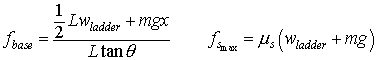## Ladder leaning against a wall

 Hey, who left that ladder leaning against the wall .. oh, well, better climb up and .. yikes it is starting to slip! I should have studied my EQUILIBRIUM formulas! You can change the masses of the ladder and the person .. the distance the person stands from the base of the latter (measured up along the ladder) - distances are measured as fraction of the length of the ladder. You can also set the coefficient of static friction underneath the ladder (the wall to the left is frictionless). Finally, you can change the lean angle of the ladder (with respect to the ground). Set the starting values :            Person weight = mg (N) =                Ladder weight = W (N) =                ---------------------------------------------------            X person (m) =     = fraction of ladder length            static friction coeff =                Theta of wire (deg) =                ---------------------------------------------------   * If you manually change values ... Note : The maximum static friction force is listed, and an arrow is shown under the base of the arrow. The force needed to keep the base of the ladder from sliding is also calculated and shown. If the Fbase is greater than Fsmax .. then the ladder will slip! But, that becomes a more complicated problem ... so I faked it .. I show an "accelerating" ladder - but treat it as only a rough approximation (and the force vectors/values are no longer any good .. but it is true that the ladder would slip!). You might find these equations useful :But, note that the X here is true distance, not fraction.